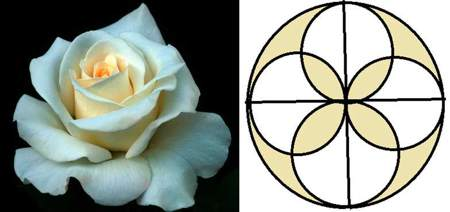# A Rose For My MotherMandy tried to draw a rose for Mother's Day using many circles. He started out with a large circle of radius 7cm, and then drew 4 smaller circles in a symmetrical way. He then colored in parts of the flower in yellow. What is the area $($in $\text{cm}^2)$ of the yellow part?

The figure is completely symmetrical.
The figure is not drawn to scale.
Take $\pi = \frac{ 22}{7}$.

×